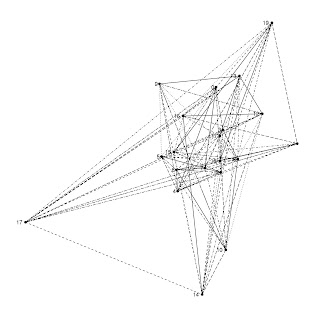Want to share your content on R-bloggers? click here if you have a blog, or here if you don't.

I have been looking around on the web and have not found anything yet related to using ggplot2 for making graphs/networks. I put together a few functions to make very simple graphs. The bipartite function especially is not ideal, as of course we only want to allow connections between unlike nodes, not all nodes. These functions do not, obviously, take full advantage of the power of ggplot2, but it’s a start.

```# ggplot network graphics functions
# g = an igraph graph object, any igraph graph object
# vplace = type of vertex placement assignment, one of rnorm, runif, etc.

gggraph <- function(g, vplace = rnorm) {

require(ggplot2)

g_ <- get.edgelist(g)
g_df <- as.data.frame(g_)
g_df\$id <- 1:length(g_df[,1])
g_df <- melt(g_df, id=3)
xy_s <- data.frame(value = unique(g_df\$value),
x = vplace(length(unique(g_df\$value))),
y = vplace(length(unique(g_df\$value))))
g_df2 <- merge(g_df, xy_s, by = "value")

p <- ggplot(g_df2, aes(x, y)) +
geom_point() +
geom_line(size = 0.3, aes(group = id, linetype = id)) +
geom_text(size = 3, hjust = 1.5, aes(label = value)) +
theme_bw() +
opts(panel.grid.major=theme_blank(),
panel.grid.minor=theme_blank(),
axis.text.x=theme_blank(),
axis.text.y=theme_blank(),
axis.title.x=theme_blank(),
axis.title.y=theme_blank(),
axis.ticks=theme_blank(),
panel.border=theme_blank(),
legend.position="none")

p

}

ggbigraph <- function(g) {

require(ggplot2)

g_ <- get.edgelist(g)
g_df <- as.data.frame(g_)
g_df\$id <- 1:length(g_df[,1])
g_df <- melt(g_df, id=3)
xy_s <- data.frame(value = unique(g_df\$value),
x = c(rep(2, length(unique(g_df\$value))/2), rep(4, length(unique(g_df\$value))/2)),
y = rep(seq(1, length(unique(g_df\$value))/2, 1), 2))
g_df2 <- merge(g_df, xy_s, by = "value")

p <- ggplot(g_df2, aes(x, y)) +
geom_point() +
geom_line(size = 0.3, aes(group = id, linetype = id)) +
geom_text(size = 3, hjust = 1.5, aes(label = value)) +
theme_bw() +
opts(panel.grid.major=theme_blank(),
panel.grid.minor=theme_blank(),
axis.text.x=theme_blank(),
axis.text.y=theme_blank(),
axis.title.x=theme_blank(),
axis.title.y=theme_blank(),
axis.ticks=theme_blank(),
panel.border=theme_blank(),
legend.position="none")

p

}

```

g <- erdos.renyi.game(20, 5, type="gnm")
```gggraph(g, rnorm)

g <- barabasi.game(20)
gggraph(g, rnorm)

g <- grg.game(20, 0.45, torus=FALSE)
gggraph(g, rnorm)

g <- growing.random.game(20, citation=FALSE)
gggraph(g, rnorm)

g <- watts.strogatz.game(1, 20, 5, 0.05)
gggraph(g, rnorm)
```# A bipartite graphs

```g <- grg.game(20, 0.45, torus=FALSE)
ggbigraph(g)
```